# Bridges SDE’s

Consider now a $$d$$-dimensional stochastic process $$X_{t}$$ defined on a probability space $$(\Omega, \mathfrak{F},\mathbb{P})$$. We say that the bridge associated to $$X_{t}$$ conditioned to the event $$\{X_{T}= a\}$$ is the process: $\{\tilde{X}_{t}, t_{0} \leq t \leq T \}=\{X_{t}, t_{0} \leq t \leq T: X_{T}= a \}$ where $$T$$ is a deterministic fixed time and $$a \in \mathbb{R}^d$$ is fixed too.

# The bridgesdekd() functions

The (S3) generic function bridgesdekd() (where k=1,2,3) for simulation of 1,2 and 3-dim bridge stochastic differential equations,Itô or Stratonovich type, with different methods. The main arguments consist:

• The drift and diffusion coefficients as R expressions that depend on the state variable x (y and z) and time variable t.
• The number of simulation steps N.
• The number of the solution trajectories to be simulated by M (default: M=1).
• Initial value x0 at initial time t0.
• Terminal value y final time T
• The integration step size Dt (default: Dt=(T-t0)/N).
• The choice of process types by the argument type="ito" for Ito or type="str" for Stratonovich (by default type="ito").
• The numerical method to be used by method (default method="euler").

By Monte-Carlo simulations, the following statistical measures (S3 method) for class bridgesdekd() (where k=1,2,3) can be approximated for the process at any time $$t \in [t_{0},T]$$ (default: at=(T-t0)/2):

• The expected value $$\text{E}(X_{t})$$ at time $$t$$, using the command mean.
• The variance $$\text{Var}(X_{t})$$ at time $$t$$, using the command moment with order=2 and center=TRUE.
• The median $$\text{Med}(X_{t})$$ at time $$t$$, using the command Median.
• The mode $$\text{Mod}(X_{t})$$ at time $$t$$, using the command Mode.
• The quartile of $$X_{t}$$ at time $$t$$, using the command quantile.
• The maximum and minimum of $$X_{t}$$ at time $$t$$, using the command min and max.
• The skewness and the kurtosis of $$X_{t}$$ at time $$t$$, using the command skewness and kurtosis.
• The coefficient of variation (relative variability) of $$X_{t}$$ at time $$t$$, using the command cv.
• The central moments up to order $$p$$ of $$X_{t}$$ at time $$t$$, using the command moment.
• The result summaries of the results of Monte-Carlo simulation at time $$t$$, using the command summary.

We can just make use of the rsdekd() function (where k=1,2,3) to build our random number for class bridgesdekd() (where k=1,2,3) at any time $$t \in [t_{0},T]$$. the main arguments consist:

• object an object inheriting from class bridgesdekd() (where k=1,2,3).
• at time between $$s=t0$$ and $$t=T$$.

The function dsde() (where k=1,2,3) approximate transition density for class bridgesdekd() (where k=1,2,3), the main arguments consist:

• object an object inheriting from class bridgesdekd() (where k=1,2,3).
• at time between $$s=t0$$ and $$t=T$$.
• pdf probability density function Joint or Marginal.

The following we explain how to use this functions.

# bridgesde1d()

Assume that we want to describe the following bridge sde in Itô form: $\begin{equation}\label{eq0166} dX_t = \frac{1-X_t}{1-t} dt + X_t dW_{t},\quad X_{t_{0}}=3 \quad\text{and}\quad X_{T}=1 \end{equation}$

We simulate a flow of $$1000$$ trajectories, with integration step size $$\Delta t = 0.001$$, and $$x_0 = 3$$ at time $$t_0 = 0$$, $$y = 1$$ at terminal time $$T=1$$.

R> f <- expression((1-x)/(1-t))
R> g <- expression(x)
R> mod <- bridgesde1d(drift=f,diffusion=g,x0=3,y=1,M=1000,method="milstein")
R> mod
Itô Bridge Sde 1D:
| dX(t) = (1 - X(t))/(1 - t) * dt + X(t) * dW(t)
Method:
| First-order Milstein scheme
Summary:
| Size of process   | N = 1001.
| Crossing realized | C = 976 among 1000.
| Initial value     | x0 = 3.
| Ending value      | y = 1.
| Time of process   | t in [0,1].
| Discretization    | Dt = 0.001.
R> summary(mod) ## default: summary at time = (T-t0)/2

Monte-Carlo Statistics for X(t) at time t = 0.5
| Crossing realized 976 among 1000

Mean               1.90914
Variance           1.38112
Median             1.62166
Mode               1.20732
First quartile     1.11634
Third quartile     2.34180
Minimum            0.28891
Maximum           11.16228
Skewness           1.95820
Kurtosis           9.42262
Coef-variation     0.61557
3th-order moment   3.17835
4th-order moment  17.97349
5th-order moment 110.45509
6th-order moment 831.50681

In Figure 1, we present the flow of trajectories, the mean path (red lines) of solution of $$X_{t}|X_{0}=3,X_{T}=1$$:

R> plot(mod,ylab=expression(X[t]))
R> lines(time(mod),apply(mod$X,1,mean),col=2,lwd=2) R> legend("topleft","mean path",inset = .01,col=2,lwd=2,cex=0.8,bty="n")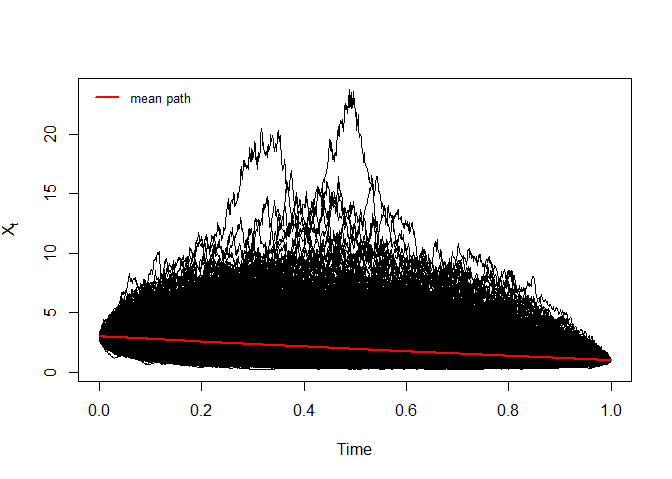Bridge sde 1D Figure 2, show approximation results for $$m(t)=\text{E}(X_{t}|X_{0}=3,X_{T}=1)$$ and $$S(t)=\text{V}(X_{t}|X_{0}=3,X_{T}=1)$$: R> m <- apply(mod$X,1,mean)
R> S  <- apply(mod$X,1,var) R> out <- data.frame(m,S) R> matplot(time(mod), out, type = "l", xlab = "time", ylab = "", col=2:3,lwd=2,lty=2:3,las=1) R> legend("topright",c(expression(m(t),S(t))),col=2:3,lty=2:3,lwd=2,bty="n")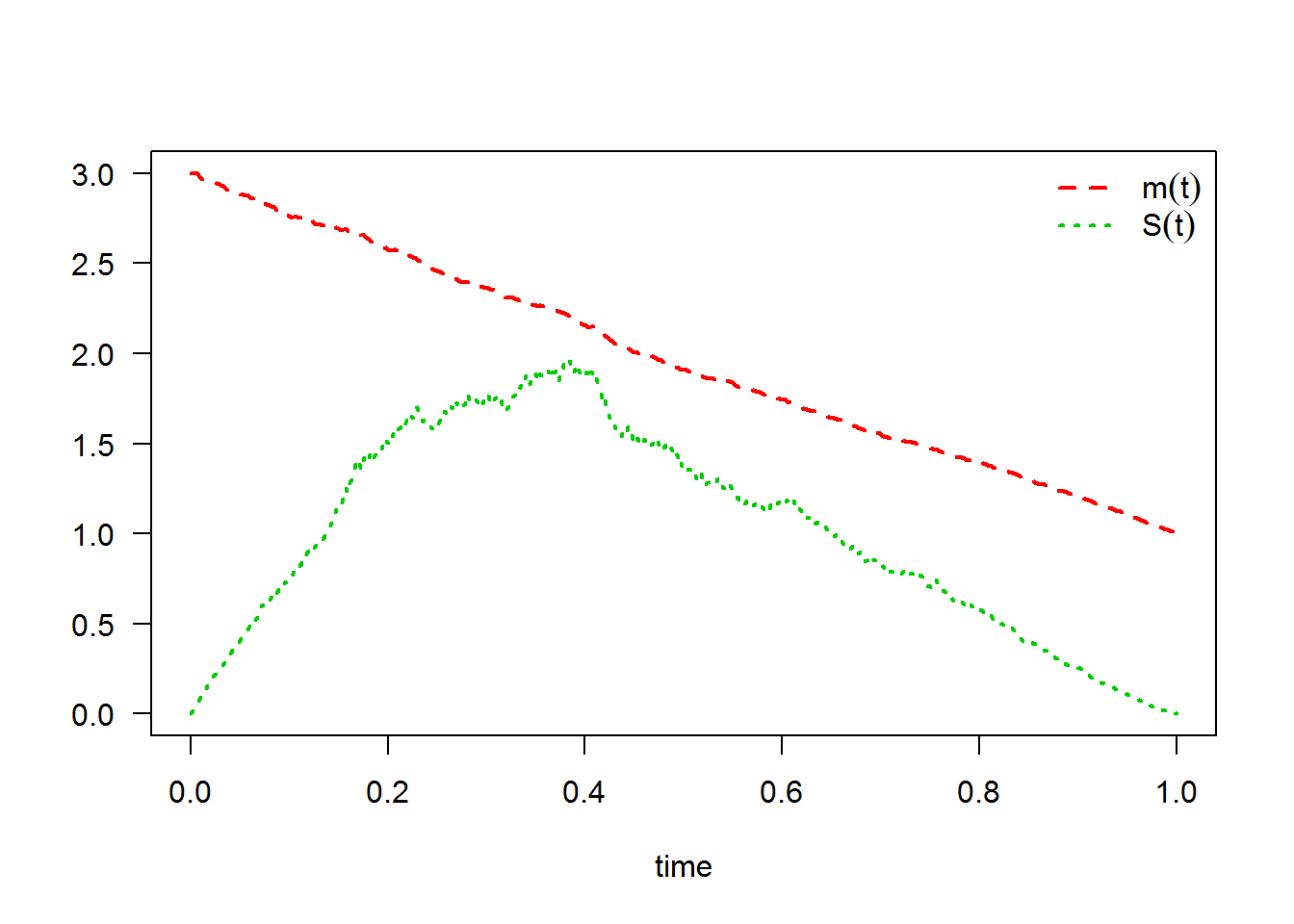The following statistical measures (S3 method) for class bridgesde1d() can be approximated for the $$X_{t}|X_{0}=3,X_{T}=1$$ process at any time $$t$$, for example at=0.55: R> s = 0.55 R> mean(mod, at = s)  1.8377 R> moment(mod, at = s , center = TRUE , order = 2) ## variance  1.2523 R> Median(mod, at = s)  1.5342 R> Mode(mod, at = s)  1.2192 R> quantile(mod , at = s)  0% 25% 50% 75% 100% 0.27505 1.06531 1.53425 2.24487 8.58112  R> kurtosis(mod , at = s)  7.1178 R> skewness(mod , at = s)  1.7527 R> cv(mod , at = s )  0.60924 R> min(mod , at = s)  0.27505 R> max(mod , at = s)  8.5811 R> moment(mod, at = s , center= TRUE , order = 4)  11.185 R> moment(mod, at = s , center= FALSE , order = 4)  66.049 The result summaries of the $$X_{t}|X_{0}=3,X_{T}=1$$ process at time $$t=0.55$$: R> summary(mod, at = 0.55)  Monte-Carlo Statistics for X(t) at time t = 0.55 | Crossing realized 976 among 1000 Mean 1.83773 Variance 1.25355 Median 1.53425 Mode 1.21915 First quartile 1.06531 Third quartile 2.24487 Minimum 0.27505 Maximum 8.58112 Skewness 1.75275 Kurtosis 7.11779 Coef-variation 0.60924 3th-order moment 2.45999 4th-order moment 11.18490 5th-order moment 48.00335 6th-order moment 240.83550 Hence we can just make use of the rsde1d() function to build our random number generator for $$X_{t}|X_{0}=3,X_{T}=1$$ at time $$t=0.55$$: R> x <- rsde1d(object = mod, at = s) R> head(x, n = 10)   0.81902 1.60237 1.31500 1.10784 1.94418 1.87228 5.22035 2.94248  1.35050 0.94817 R> summary(x)  Min. 1st Qu. Median Mean 3rd Qu. Max. 0.275 1.065 1.534 1.838 2.245 8.581  Display the random number generator for $$X_{t}|X_{0}=3,X_{T}=1$$, see Figure 3: R> plot(time(mod),mod$X[,1],type="l",ylab="X(t)",xlab="time",axes=F,lty=3)
R> points(s,x,pch=19,col=2,cex=0.5)
R> lines(c(s,s),c(0,x),lty=2,col=2)
R> lines(c(0,s),c(x,x),lty=2,col=2)
R> axis(1, s, bquote(at==.(s)),col=2,col.ticks=2)
R> axis(2, x, bquote(X[t==.(s)]),col=2,col.ticks=2)
R> legend('topright',col=2,pch=19,legend=bquote(X[t==.(s)]==.(x)),bty = 'n')
R> box()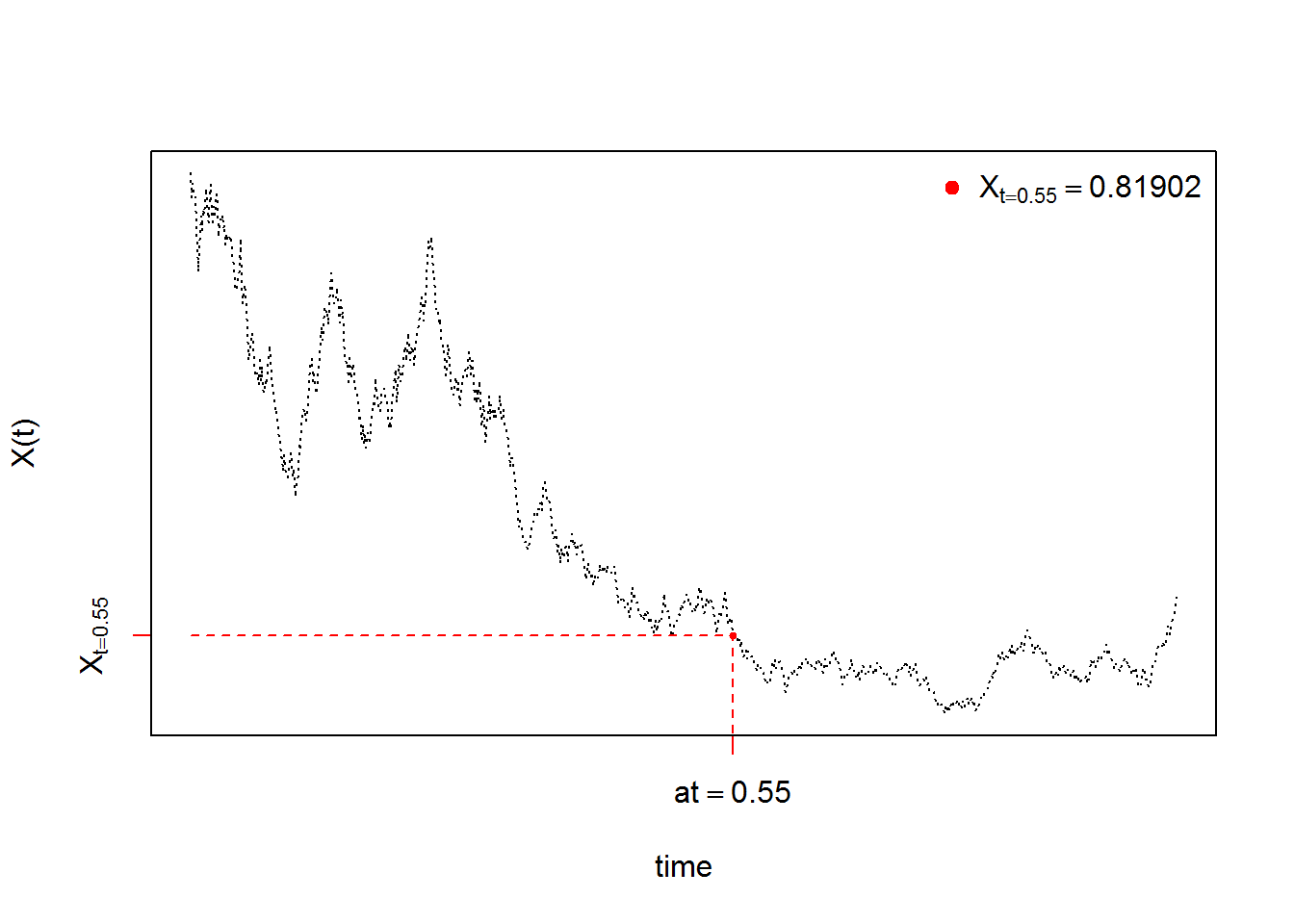The function dsde1d() can be used to show the kernel density estimation for $$X_{t}|X_{0}=3,X_{T}=1$$ at time $$t=0.55$$ (hist=TRUE based on truehist() function in MASS package):

R> dens <- dsde1d(mod, at = s)
R> dens

Density of X(t-t0)|X(t0) = 3, X(T) = 1 at time t = 0.55

Data: x (976 obs.); Bandwidth 'bw' = 0.2

x                f(x)
Min.   :-0.3249   Min.   :0.00002
1st Qu.: 2.0516   1st Qu.:0.00195
Median : 4.4281   Median :0.01614
Mean   : 4.4281   Mean   :0.10509
3rd Qu.: 6.8046   3rd Qu.:0.13109
Max.   : 9.1810   Max.   :0.54296  
R> plot(dens,hist=TRUE) ## histgramme
R> plot(dens,add=TRUE)  ## kernel density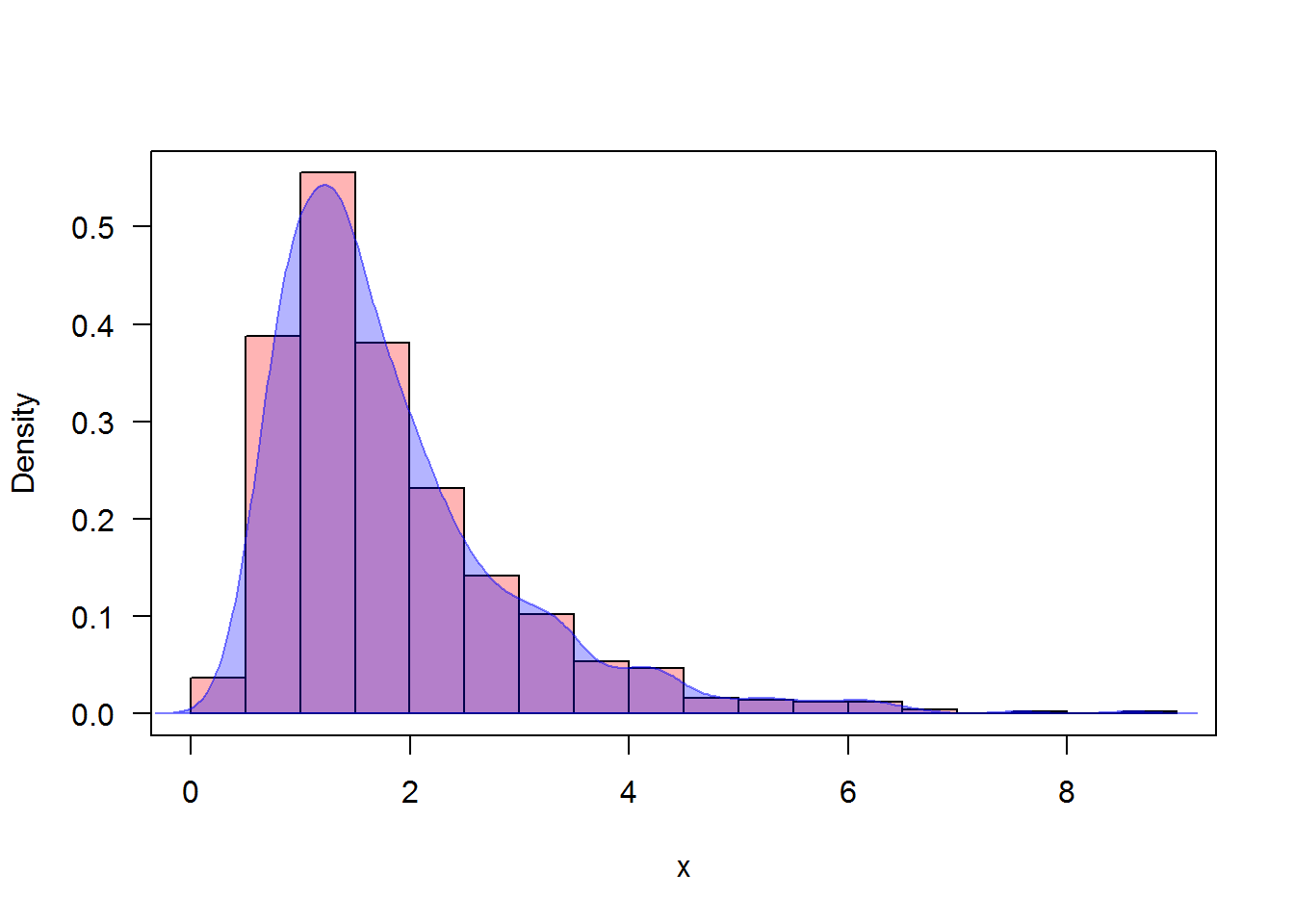Approximate the transitional densitie of $$X_{t}|X_{0}=3,X_{T}=1$$ at $$t-s = \{0.25,0.75\}$$:

R> plot(dsde1d(mod,at=0.75))
R> legend('topright',col=c('#0000FF4B','#FF00004B'),pch=15,legend=c("t-s=0.25","t-s=0.75"),bty = 'n')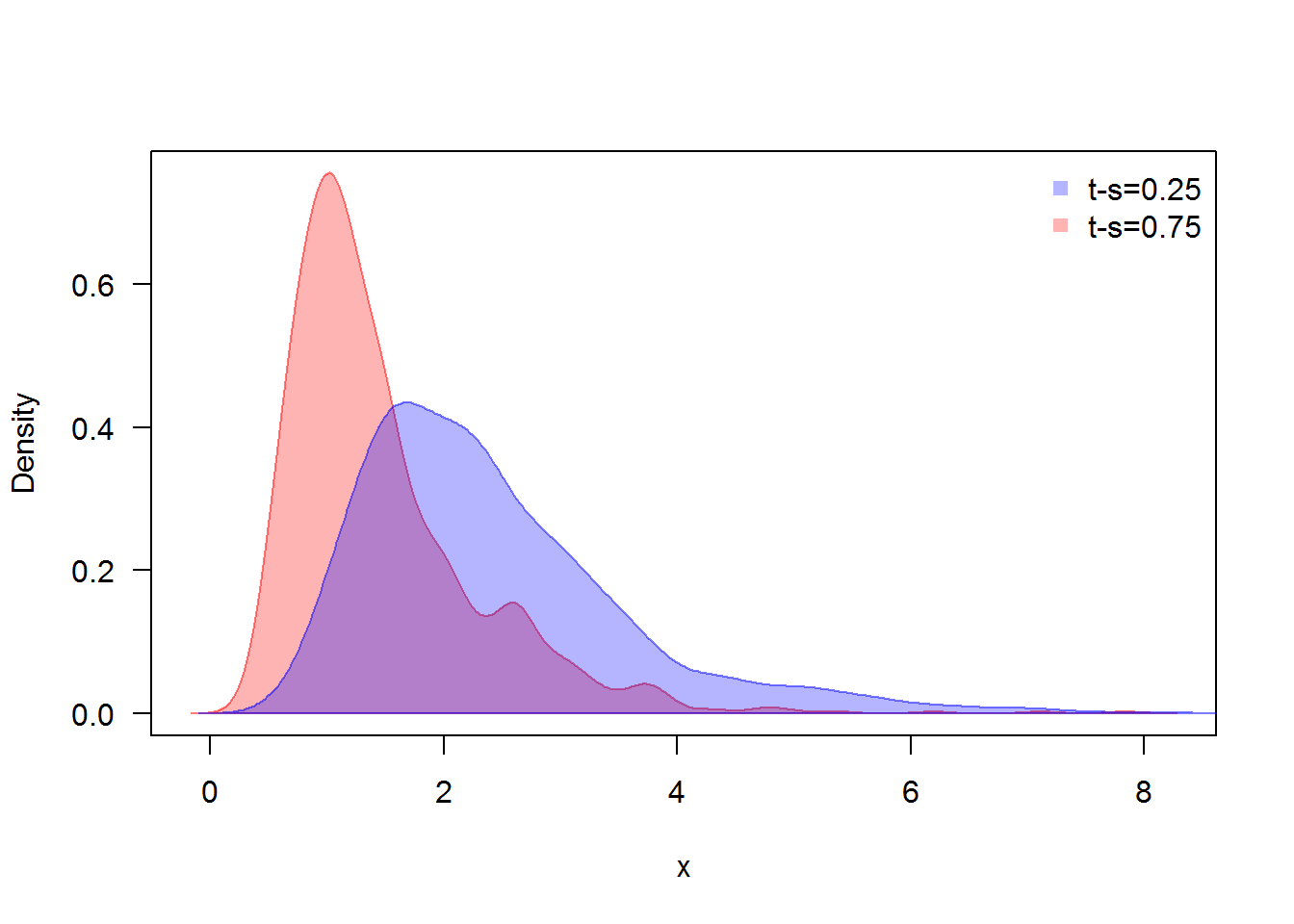Transitional densitie at time $$t-s = 0.25,0.75$$

# bridgesde2d()

Assume that we want to describe the following $$2$$-dimensional bridge SDE’s in Stratonovich form:

$\begin{equation}\label{eq:09} \begin{cases} dX_t = -(1+Y_{t}) X_{t} dt + 0.2 (1-Y_{t})\circ dW_{1,t},\quad X_{t_{0}}=1 \quad\text{and}\quad X_{T}=1\\ dY_t = -(1+X_{t}) Y_{t} dt + 0.1 (1-X_{t}) \circ dW_{2,t},\quad Y_{t_{0}}=-0.5 \quad\text{and}\quad Y_{T}=0.5 \end{cases} \end{equation}$

We simulate a flow of $$1000$$ trajectories, with integration step size $$\Delta t = 0.01$$, and using Runge-Kutta method order 1:

R> fx <- expression(-(1+y)*x , -(1+x)*y)
R> gx <- expression(0.2*(1-y),0.1*(1-x))
R> mod2 <- bridgesde2d(drift=fx,diffusion=gx,x0=c(1,-0.5),y=c(1,0.5),Dt=0.01,M=1000,type="str",method="rk1")
R> mod2
Stratonovich Bridge Sde 2D:
| dX(t) = -(1 + Y(t)) * X(t) * dt + 0.2 * (1 - Y(t)) o dW1(t)
| dY(t) = -(1 + X(t)) * Y(t) * dt + 0.1 * (1 - X(t)) o dW2(t)
Method:
| Runge-Kutta method with order 1
Summary:
| Size of process   | N = 1001.
| Crossing realized | C = 1000 among 1000.
| Initial values    | x0 = (1,-0.5).
| Ending values     | y = (1,0.5).
| Time of process   | t in [0,10].
| Discretization    | Dt = 0.01.
R> summary(mod2) ## default: summary at time = (T-t0)/2

Monte-Carlo Statistics for (X(t),Y(t)) at time t = 5
| Crossing realized 1000 among 1000
X         Y
Mean              0.00823  -0.00137
Variance          0.02045   0.00550
Median            0.00504  -0.00246
Mode              0.02681  -0.00341
First quartile   -0.09028  -0.05356
Third quartile    0.10170   0.04916
Minimum          -0.47935  -0.23975
Maximum           0.40811   0.26743
Skewness          0.07063   0.14700
Kurtosis          2.92232   3.04759
Coef-variation   17.38261 -54.19888
3th-order moment  0.00021   0.00006
4th-order moment  0.00122   0.00009
5th-order moment  0.00000   0.00000
6th-order moment  0.00011   0.00000

In Figure 6, we present the flow of trajectories of $$X_{t}|X_{0}=1,X_{T}=1$$ and $$Y_{t}|Y_{0}=-0.5,Y_{T}=0.5$$:

R> plot(mod2,col=c('#FF00004B','#0000FF82'))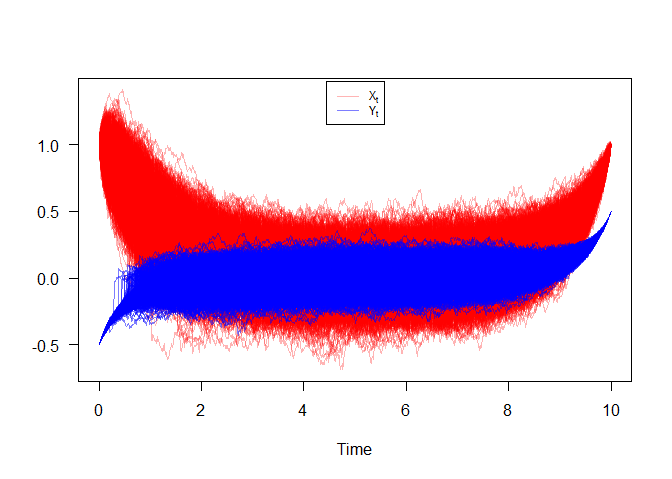Bridge sde 2D

Figure 7, show approximation results for $$m_{1}(t)=\text{E}(X_{t}|X_{0}=1,X_{T}=1)$$, $$m_{2}(t)=\text{E}(Y_{t}|Y_{0}=-0.5,Y_{T}=0.5)$$,and $$S_{1}(t)=\text{V}(X_{t}|X_{0}=1,X_{T}=1)$$, $$S_{2}(t)=\text{V}(Y_{t}|Y_{0}=-0.5,Y_{T}=0.5)$$, and $$C_{12}(t)=\text{COV}(X_{t},Y_{t}|X_{0}=1,Y_{0}=-0.5,X_{T}=1,Y_{T}=0.5)$$:

R> m1  <- apply(mod2$X,1,mean) R> m2 <- apply(mod2$Y,1,mean)
R> S1  <- apply(mod2$X,1,var) R> S2 <- apply(mod2$Y,1,var)
R> C12 <- sapply(1:dim(mod2$X),function(i) cov(mod2$X[i,],mod2$Y[i,])) R> out2 <- data.frame(m1,m2,S1,S2,C12) R> matplot(time(mod2), out2, type = "l", xlab = "time", ylab = "", col=2:6,lwd=2,lty=2:6,las=1) R> legend("top",c(expression(m(t),m(t),S(t),S(t),C(t))),col=2:6,lty=2:6,lwd=2,bty="n")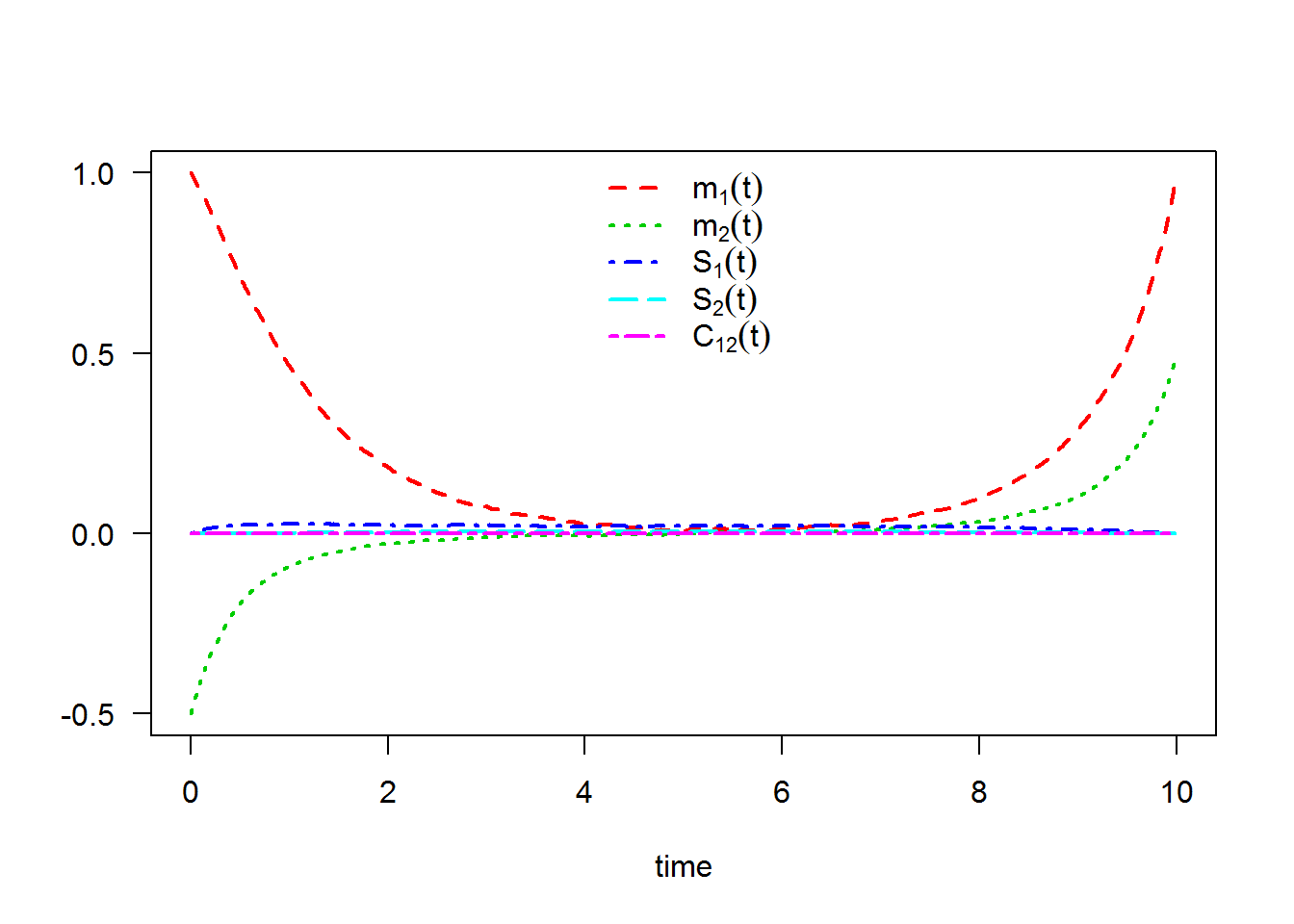The following statistical measures (S3 method) for class bridgesde2d() can be approximated for the $$X_{t}|X_{0}=1,X_{T}=1$$ and $$Y_{t}|Y_{0}=-0.5,Y_{T}=0.5$$ process at any time $$t$$, for example at=6.75: R> s = 6.75 R> mean(mod2, at = s)  0.0243721 0.0065202 R> moment(mod2, at = s , center = TRUE , order = 2) ## variance  0.0197170 0.0046843 R> Median(mod2, at = s)  0.0320785 0.0072444 R> Mode(mod2, at = s)  0.0524349 0.0025239 R> quantile(mod2 , at = s) $x
0%       25%       50%       75%      100%
-0.436010 -0.066898  0.032078  0.111749  0.615650

$y 0% 25% 50% 75% 100% -0.2922471 -0.0368836 0.0072444 0.0526186 0.2333810  R> kurtosis(mod2 , at = s)  3.3321 3.4421 R> skewness(mod2 , at = s)  -0.001584 -0.092377 R> cv(mod2 , at = s )  5.7643 10.5021 R> min(mod2 , at = s)  -0.43601 -0.29225 R> max(mod2 , at = s)  0.61565 0.23338 R> moment(mod2 , at = s , center= TRUE , order = 4)  0.00129800 0.00007568 R> moment(mod2 , at = s , center= FALSE , order = 4)  0.001368200 0.000076103 The result summaries of the $$X_{t}|X_{0}=1,X_{T}=1$$ and $$Y_{t}|Y_{0}=-0.5,Y_{T}=0.5$$ process at time $$t=6.75$$: R> summary(mod2, at = 6.75)  Monte-Carlo Statistics for (X(t),Y(t)) at time t = 6.75 | Crossing realized 1000 among 1000 X Y Mean 0.02437 0.00652 Variance 0.01974 0.00469 Median 0.03208 0.00724 Mode 0.05243 0.00252 First quartile -0.06690 -0.03688 Third quartile 0.11175 0.05262 Minimum -0.43601 -0.29225 Maximum 0.61565 0.23338 Skewness -0.00158 -0.09238 Kurtosis 3.33215 3.44207 Coef-variation 5.76427 10.50213 3th-order moment 0.00000 -0.00003 4th-order moment 0.00130 0.00008 5th-order moment 0.00004 0.00000 6th-order moment 0.00016 0.00000 Hence we can just make use of the rsde2d() function to build our random number generator for the couple $$X_{t},Y_{t}|X_{0}=1,Y_{0}=-0.5,X_{T}=1,Y_{T}=0.5$$ at time $$t=6.75$$: R> x2 <- rsde2d(object = mod2, at = s) R> head(x2, n = 10)  x y 1 -0.081996 0.1575094 2 0.033092 0.1516347 3 0.111655 -0.0730191 4 0.035723 0.1342599 5 0.046617 0.0422785 6 -0.077607 -0.0307018 7 -0.236959 0.1109109 8 -0.139944 -0.0294634 9 0.052166 0.0034076 10 0.057642 0.0576556 R> summary(x2)  x y Min. :-0.4360 Min. :-0.29225 1st Qu.:-0.0669 1st Qu.:-0.03688 Median : 0.0321 Median : 0.00724 Mean : 0.0244 Mean : 0.00652 3rd Qu.: 0.1118 3rd Qu.: 0.05262 Max. : 0.6157 Max. : 0.23338  Display the random number generator for the couple $$X_{t},Y_{t}|X_{0}=1,Y_{0}=-0.5,X_{T}=1,Y_{T}=0.5$$, see Figure 8: R> plot(ts.union(mod2$X[,1],mod2$Y[,1]),col=1:2,lty=3,plot.type="single",type="l",ylab= "",xlab="time",axes=F) R> points(s,x2$x,pch=19,col=3,cex=0.8)
R> points(s,x2$y,pch=19,col=4,cex=0.8) R> lines(c(s,s),c(-10,x2$x),lty=2,col=6)
R> lines(c(0,s),c(x2$x,x2$x),lty=2,col=3)
R> lines(c(0,s),c(x2$y,x2$y),lty=2,col=4)
R> axis(1, s, bquote(at==.(s)),col=6,col.ticks=6)
R> axis(2, x2$x, bquote(X[t==.(s)]),col=3,col.ticks=3) R> axis(2, x2$y, bquote(Y[t==.(s)]),col=4,col.ticks=4)
R> legend('topright',legend=bquote(c(X[t==.(s)]==.(x2$x),Y[t==.(s)]==.(x2$y))),bty = 'n')
R> box()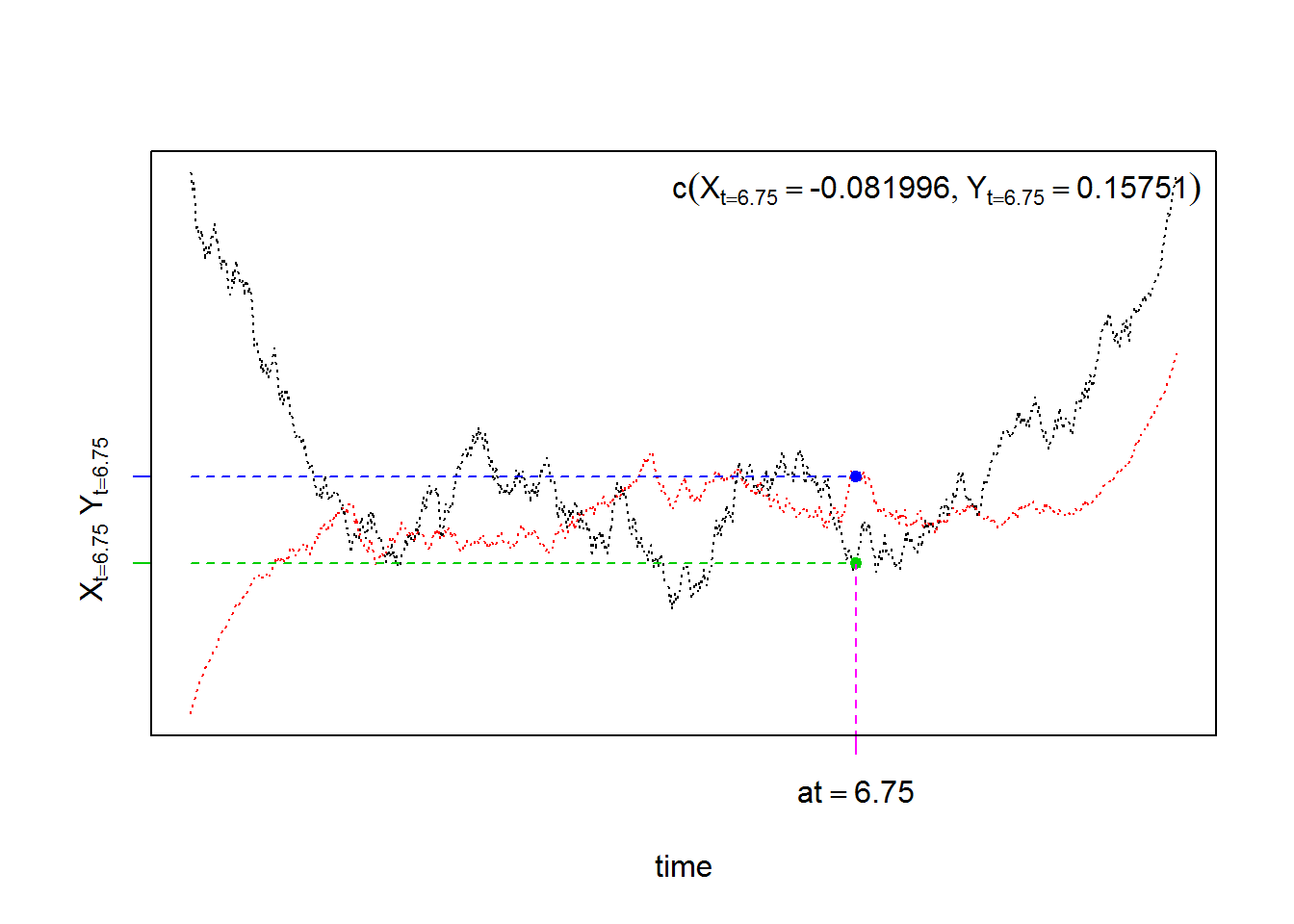For each SDE type and for each numerical scheme, the density of $$X_{t}|X_{0}=1,X_{T}=1$$ and $$Y_{t}|Y_{0}=-0.5,Y_{T}=0.5$$ at time $$t=6.75$$ are reported using dsde2d() function, see e.g. Figure 9:

R> denM <- dsde2d(mod2,pdf="M",at =s)
R> denM

Marginal density of X(t-t0)|X(t0) = 1, X(T) = 1 at time t = 6.75

Data: x (1000 obs.);    Bandwidth 'bw' = 0.03014

x                 f(x)
Min.   :-0.52643   Min.   :0.00015
1st Qu.:-0.21830   1st Qu.:0.01714
Median : 0.08982   Median :0.25895
Mean   : 0.08982   Mean   :0.81057
3rd Qu.: 0.39794   3rd Qu.:1.55619
Max.   : 0.70607   Max.   :2.96729

Marginal density of Y(t-t0)|Y(t0) = -0.5, Y(T) = 0.5 at time t = 6.75

Data: y (1000 obs.);    Bandwidth 'bw' = 0.0151

y                 f(y)
Min.   :-0.33755   Min.   :0.0003
1st Qu.:-0.18349   1st Qu.:0.0280
Median :-0.02943   Median :0.4257
Mean   :-0.02943   Mean   :1.6212
3rd Qu.: 0.12462   3rd Qu.:2.9782
Max.   : 0.27868   Max.   :6.1824  
R> plot(denM, main="Marginal Density")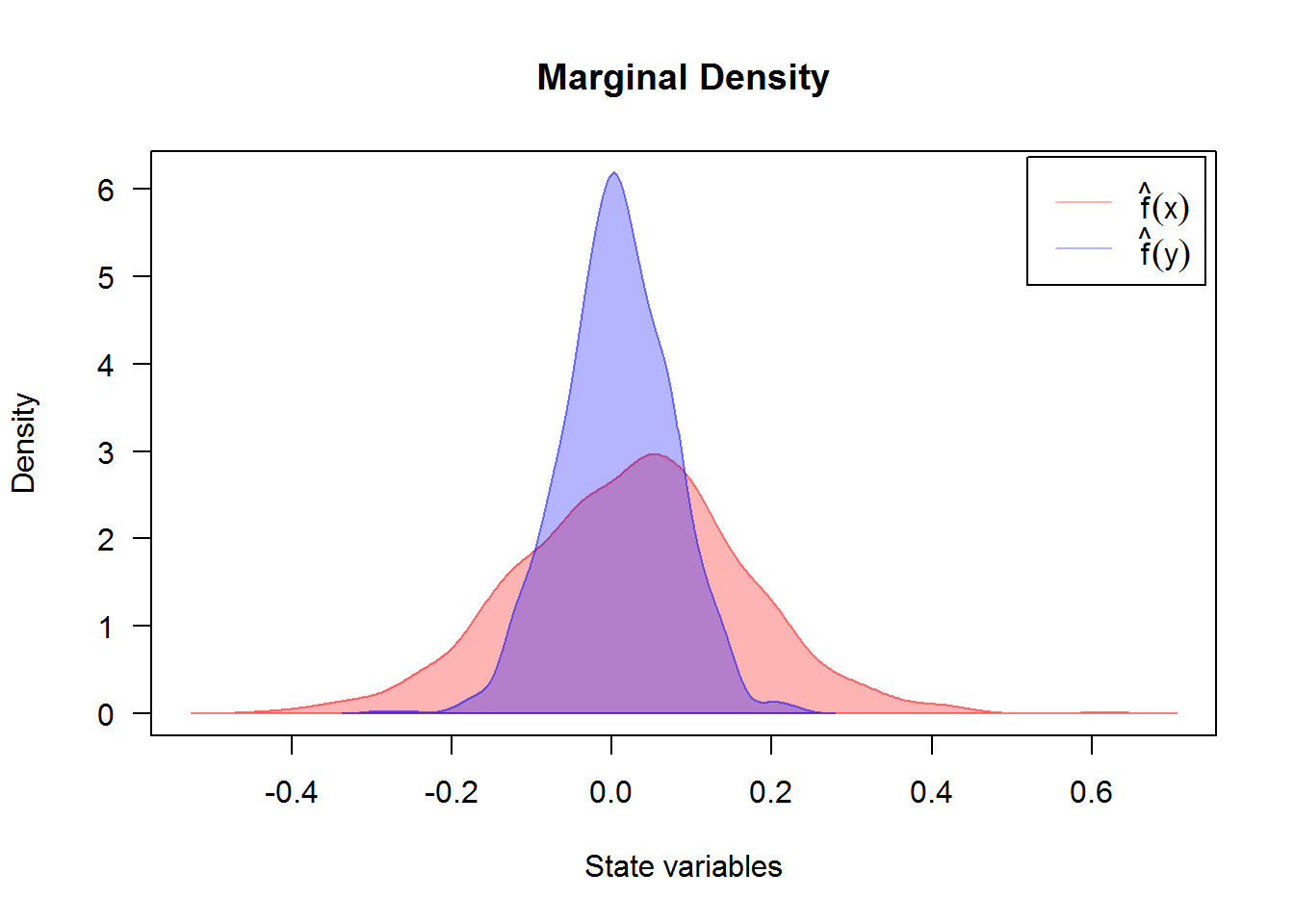Created using dsde2d() plotted in (x, y)-space with dim = 2. A contour and image plot of density obtained from a realization of the couple $$X_{t},Y_{t}|X_{0}=1,Y_{0}=-0.5,X_{T}=1,Y_{T}=0.5$$ at time t=6.75.

R> denJ <- dsde2d(mod2, pdf="J", n=100,at =s)
R> denJ

Joint density of (X(t-t0),Y(t-t0)|X(t0)=1,Y(t0)=-0.5,X(T)=1,Y(T)=0.5) at time t = 6.75

Data: (x,y) (2 x 1000 obs.);

x                  y                 f(x,y)
Min.   :-0.43601   Min.   :-0.292247   Min.   : 0.0000
1st Qu.:-0.17310   1st Qu.:-0.160840   1st Qu.: 0.0016
Median : 0.08982   Median :-0.029433   Median : 0.2135
Mean   : 0.08982   Mean   :-0.029433   Mean   : 1.7686
3rd Qu.: 0.35273   3rd Qu.: 0.101974   3rd Qu.: 1.6754
Max.   : 0.61565   Max.   : 0.233381   Max.   :18.9834  
R> plot(denJ,display="contour",main="Bivariate Transition Density at time t=6.755")
R> plot(denJ,display="image",main="Bivariate Transition Density at time t=6.755")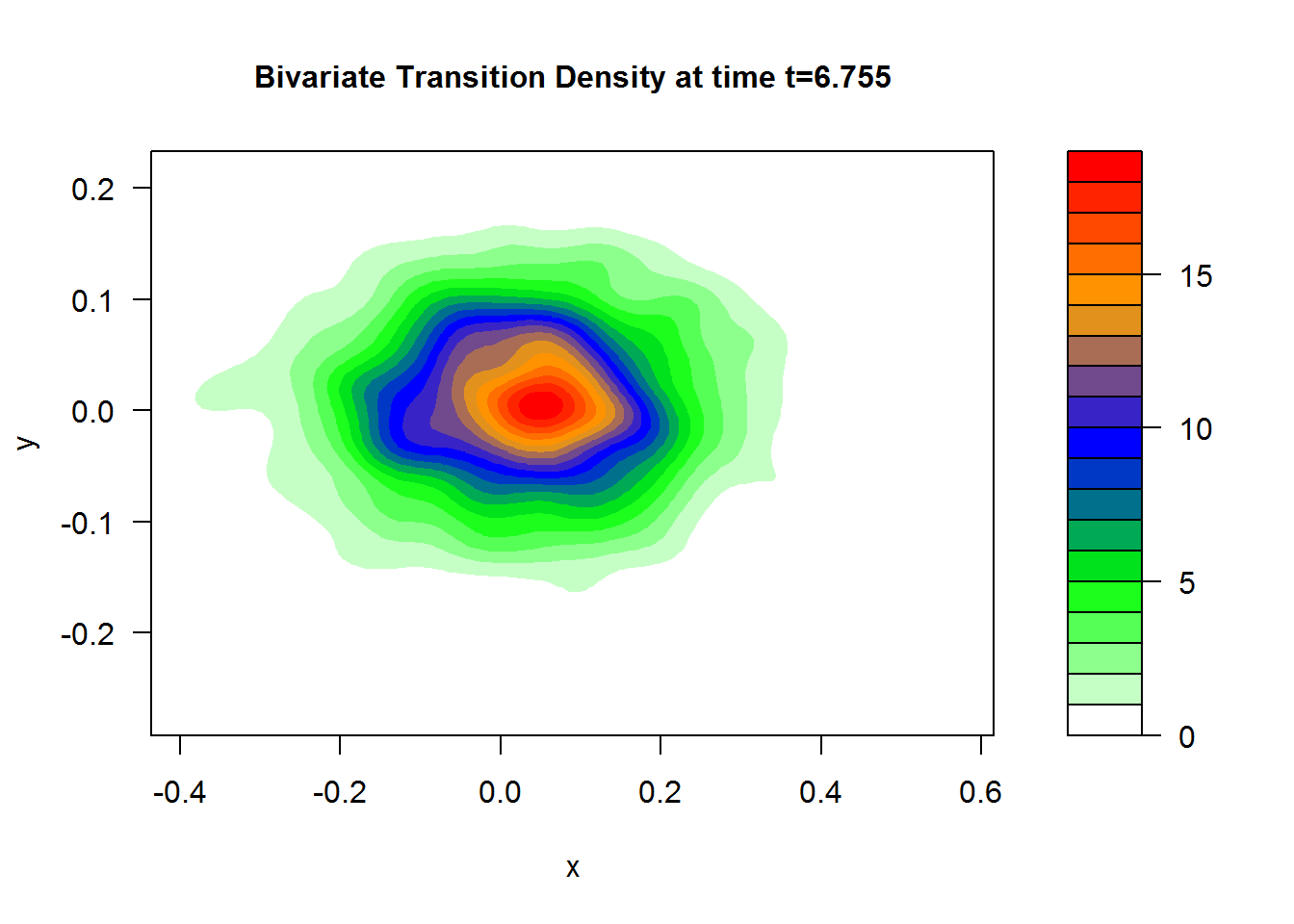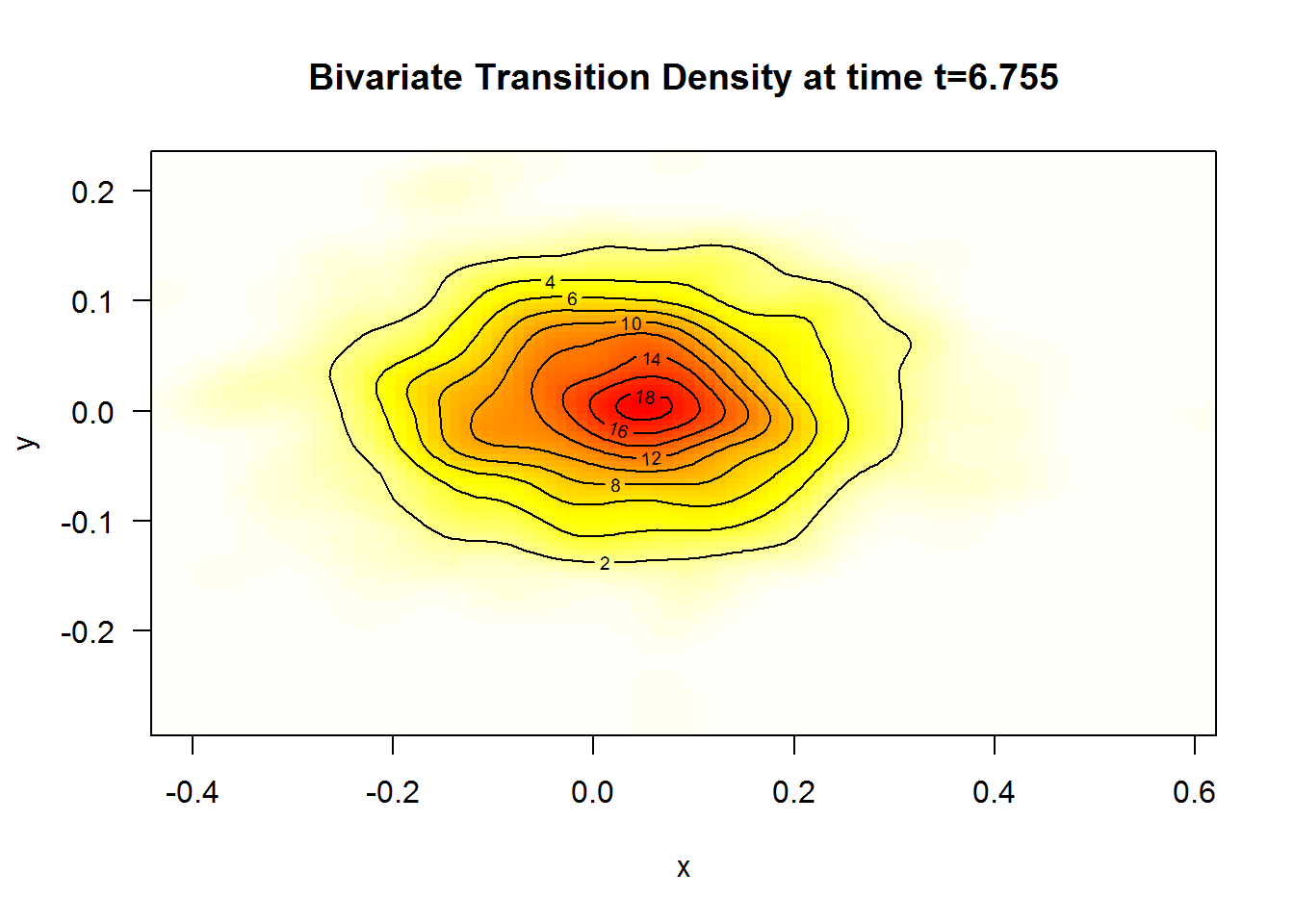A $$3$$D plot of the transition density at $$t=6.75$$ obtained with:

R> plot(denJ,main="Bivariate Transition Density at time t=6.75")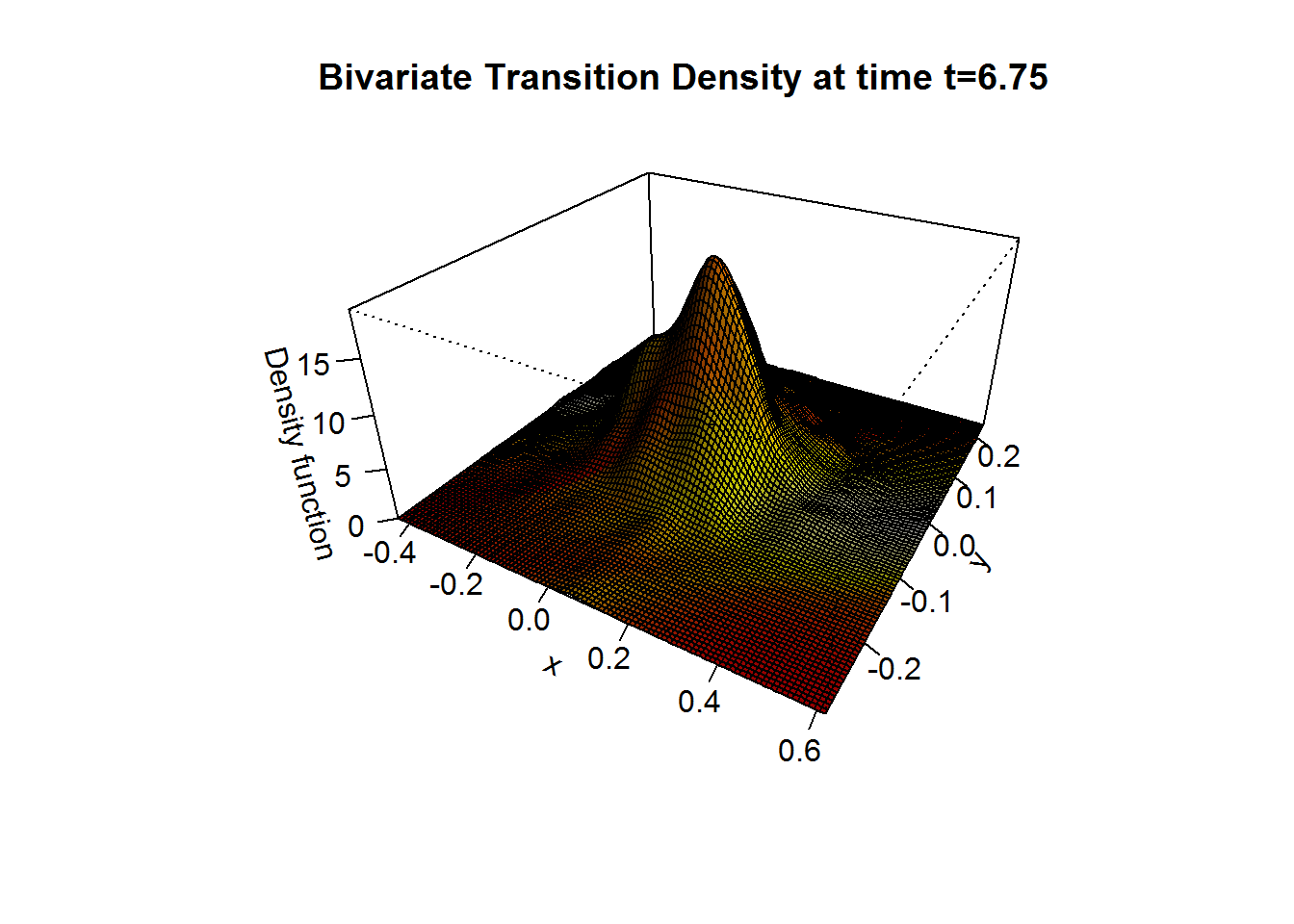We approximate the bivariate transition density over the set transition horizons $$t\in [1,9]$$ with $$\Delta t = 0.005$$ using the code:

R> for (i in seq(1,9,by=0.005)){
+ plot(dsde2d(mod2, at = i,n=100),display="contour",main=paste0('Transition Density \n t = ',i))
+ }

# bridgesde3d()

Assume that we want to describe the following bridges SDE’s (3D) in Itô form:

$\begin{equation} \begin{cases} dX_t = -4 (1+X_{t}) Y_{t} dt + 0.2 dW_{1,t},\quad X_{t_{0}}=0 \quad\text{and}\quad X_{T}=0\\ dY_t = 4 (1-Y_{t}) X_{t} dt + 0.2 dW_{2,t},\quad Y_{t_{0}}=-1 \quad\text{and}\quad Y_{T}=-2\\ dZ_t = 4 (1-Z_{t}) Y_{t} dt + 0.2 dW_{3,t},\quad Z_{t_{0}}=0.5 \quad\text{and}\quad Z_{T}=0.5 \end{cases} \end{equation}$

We simulate a flow of $$1000$$ trajectories, with integration step size $$\Delta t = 0.001$$.

R> fx <- expression(-4*(1+x)*y, 4*(1-y)*x, 4*(1-z)*y)
R> gx <- rep(expression(0.2),3)
R> mod3 <- bridgesde3d(x0=c(0,-1,0.5),y=c(0,-2,0.5),drift=fx,diffusion=gx,M=1000)
R> mod3
Itô Bridge Sde 3D:
| dX(t) = -4 * (1 + X(t)) * Y(t) * dt + 0.2 * dW1(t)
| dY(t) = 4 * (1 - Y(t)) * X(t) * dt + 0.2 * dW2(t)
| dZ(t) = 4 * (1 - Z(t)) * Y(t) * dt + 0.2 * dW3(t)
Method:
| Euler scheme with order 0.5
Summary:
| Size of process   | N = 1001.
| Crossing realized | C = 999 among 1000.
| Initial values    | x0 = (0,-1,0.5).
| Ending values     | y  = (0,-2,0.5).
| Time of process   | t in [0,1].
| Discretization    | Dt = 0.001.
R> summary(mod3) ## default: summary at time = (T-t0)/2

Monte-Carlo Statistics for (X(t),Y(t),Z(t)) at time t = 0.5
| Crossing realized 999 among 1000
X       Y        Z
Mean              0.68414 0.50941  0.13613
Variance          0.01216 0.00688  0.01937
Median            0.68925 0.50534  0.13436
Mode              0.69037 0.49087  0.14578
First quartile    0.61579 0.45449  0.04739
Third quartile    0.75758 0.56147  0.22190
Minimum           0.27845 0.20595 -0.30892
Maximum           1.03044 0.89980  0.55761
Skewness         -0.25815 0.17550  0.09552
Kurtosis          3.40669 3.47880  3.16180
Coef-variation    0.16120 0.16286  1.02245
3th-order moment -0.00035 0.00010  0.00026
4th-order moment  0.00050 0.00016  0.00119
5th-order moment -0.00005 0.00001  0.00006
6th-order moment  0.00004 0.00001  0.00012

For plotting (back in time) using the command plot, and plot3D in space the results of the simulation are shown in Figure 12:

R> plot(mod3) ## in time
R> plot3D(mod3,display = "persp",main="3D Bridge SDE's") ## in space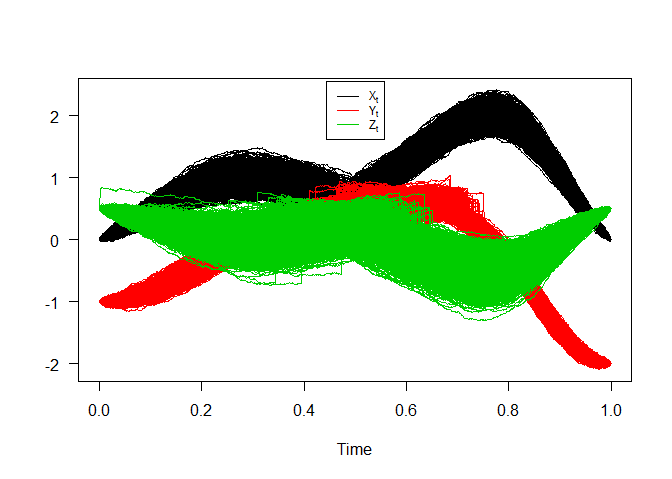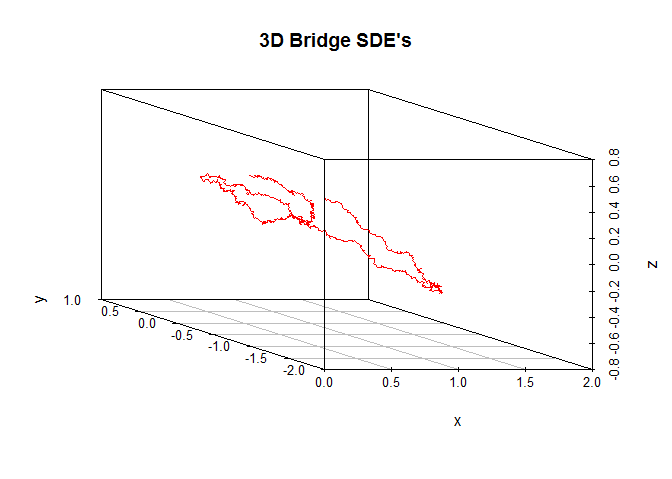Bridge sde 3D

Figure 13, show approximation results for $$m_{1}(t)=\text{E}(X_{t}|X_{0}=0,X_{T}=0)$$, $$m_{2}(t)=\text{E}(Y_{t}|Y_{0}=-1,Y_{T}=-2)$$, $$m_{3}(t)=\text{E}(Z_{t}|Z_{0}=0.5,Z_{T}=0.5)$$ and $$S_{1}(t)=\text{V}(X_{t}|X_{0}=0,X_{T}=0)$$, $$S_{2}(t)=\text{V}(Y_{t}|Y_{0}=-1,Y_{T}=-2)$$, $$S_{3}(t)=\text{V}(Z_{t}|Z_{0}=0.5,Z_{T}=0.5)$$,

R> m1  <- apply(mod3$X,1,mean) R> m2 <- apply(mod3$Y,1,mean)
R> m3  <- apply(mod3$Z,1,mean) R> S1 <- apply(mod3$X,1,var)
R> S2  <- apply(mod3$Y,1,var) R> S3 <- apply(mod3$Z,1,var)
R> out3 <- data.frame(m1,m2,m3,S1,S2,S3)
R> matplot(time(mod3), out3, type = "l", xlab = "time", ylab = "", col=2:7,lwd=2,lty=2:7,las=1)
R> legend("bottom",c(expression(m(t),m(t),m(t),S(t),S(t),S(t))),col=2:7,lty=2:7,lwd=2,bty="n")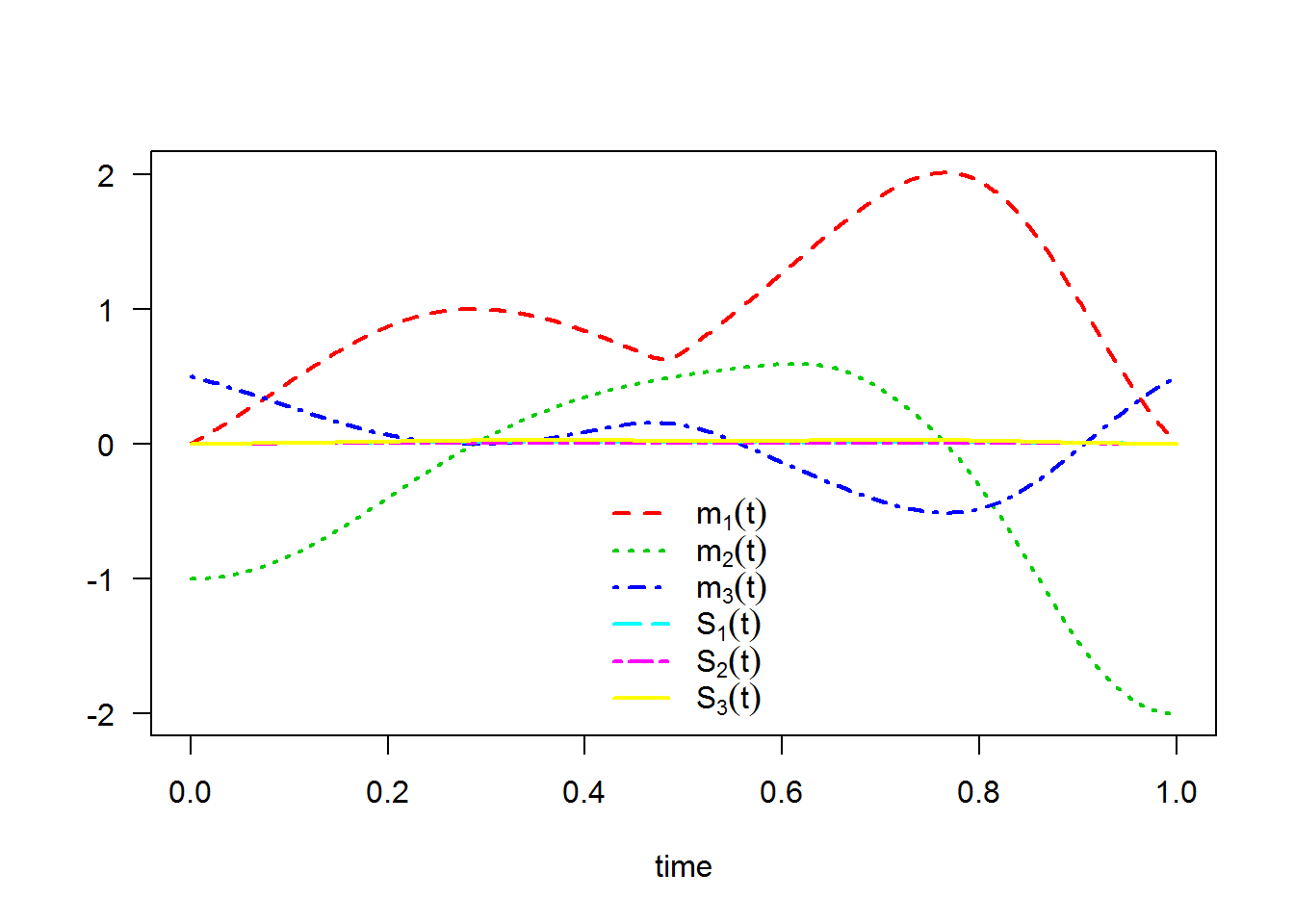The following statistical measures (S3 method) for class bridgesde3d() can be approximated for the $$X_{t}|X_{0}=0,X_{T}=0$$, $$Y_{t}|Y_{0}=-1,Y_{T}=-2$$ and $$Z_{t}|Z_{0}=0.5,Z_{T}=0.5$$ process at any time $$t$$, for example at=0.75:

R> s = 0.75
R> mean(mod3, at = s)
  2.00018  0.12203 -0.50481
R> moment(mod3, at = s , center = TRUE , order = 2) ## variance
 0.0117080 0.0045708 0.0279504
R> Median(mod3, at = s)
  1.99852  0.12505 -0.50533
R> Mode(mod3, at = s)
  1.96785  0.12733 -0.49737
R> quantile(mod3 , at = s)
$x 0% 25% 50% 75% 100% 1.6510 1.9289 1.9985 2.0769 2.3215$y
0%       25%       50%       75%      100%
-0.093024  0.075556  0.125047  0.168452  0.372778

$z 0% 25% 50% 75% 100% -1.03339 -0.62108 -0.50533 -0.39292 -0.02193  R> kurtosis(mod3 , at = s)  2.8919 2.9683 2.9435 R> skewness(mod3 , at = s)  -0.0029051 -0.0403912 -0.0325714 R> cv(mod3 , at = s )  0.054124 0.554313 -0.331351 R> min(mod3 , at = s)  1.651019 -0.093024 -1.033386 R> max(mod3 , at = s)  2.32146 0.37278 -0.02193 R> moment(mod3 , at = s , center= TRUE , order = 4)  0.000397208 0.000062141 0.002304116 R> moment(mod3 , at = s , center= FALSE , order = 4)  16.28709184 0.00068616 0.11028471 The result summaries of the $$X_{t}|X_{0}=0,X_{T}=0$$, $$Y_{t}|Y_{0}=-1,Y_{T}=-2$$ and $$Z_{t}|Z_{0}=0.5,Z_{T}=0.5$$ process at time $$t=0.75$$: R> summary(mod3, at = 0.75)  Monte-Carlo Statistics for (X(t),Y(t),Z(t)) at time t = 0.75 | Crossing realized 999 among 1000 X Y Z Mean 2.00018 0.12203 -0.50481 Variance 0.01172 0.00458 0.02798 Median 1.99852 0.12505 -0.50533 Mode 1.96785 0.12733 -0.49737 First quartile 1.92892 0.07556 -0.62108 Third quartile 2.07691 0.16845 -0.39292 Minimum 1.65102 -0.09302 -1.03339 Maximum 2.32146 0.37278 -0.02193 Skewness -0.00291 -0.04039 -0.03257 Kurtosis 2.89191 2.96834 2.94345 Coef-variation 0.05412 0.55431 -0.33135 3th-order moment 0.00000 -0.00001 -0.00015 4th-order moment 0.00040 0.00006 0.00230 5th-order moment 0.00000 0.00000 -0.00006 6th-order moment 0.00002 0.00000 0.00030 Hence we can just make use of the rsde3d() function to build our random number generator for the triplet $$X_{t},Y_{t},Z_{t}|X_{0}=0,Y_{0}=-1,Z_{0}=0.5,X_{T}=0,Y_{T}=-2,Z_{T}=0.5$$ at time $$t=0.75$$: R> x3 <- rsde3d(object = mod3, at = s) R> head(x3, n = 10)  x y z 1 1.9276 0.107201 -0.70247 2 1.8153 0.169506 -0.55594 3 2.0021 0.161518 -0.23240 4 2.0680 0.118872 -0.55609 5 2.1033 0.059167 -0.35158 6 2.1745 0.107432 -0.51440 7 1.9526 0.122690 -0.35737 8 2.0348 0.200237 -0.29961 9 2.1905 0.040172 -0.60249 10 2.0907 0.073460 -0.48571 R> summary(x3)  x y z Min. :1.65 Min. :-0.0930 Min. :-1.0334 1st Qu.:1.93 1st Qu.: 0.0756 1st Qu.:-0.6211 Median :2.00 Median : 0.1250 Median :-0.5053 Mean :2.00 Mean : 0.1220 Mean :-0.5048 3rd Qu.:2.08 3rd Qu.: 0.1684 3rd Qu.:-0.3929 Max. :2.32 Max. : 0.3728 Max. :-0.0219  Display the random number generator for triplet $$X_{t},Y_{t},Z_{t}|X_{0}=0,Y_{0}=-1,Z_{0}=0.5,X_{T}=0,Y_{T}=-2,Z_{T}=0.5$$ at time $$t=0.75$$: , see Figure 14: R> plot(ts.union(mod3$X[,1],mod3$Y[,1],mod3$Z[,1]),col=1:3,lty=3,plot.type="single",type="l",ylab= "",xlab="time",axes=F)
R> points(s,x3$x,pch=19,col=4,cex=0.8) R> points(s,x3$y,pch=19,col=5,cex=0.8)
R> points(s,x3$z,pch=19,col=6,cex=0.8) R> lines(c(s,s),c(-10,x3$x),lty=2,col=7)
R> lines(c(0,s),c(x3$x,x3$x),lty=2,col=4)
R> lines(c(0,s),c(x3$y,x3$y),lty=2,col=5)
R> lines(c(0,s),c(x3$z,x3$z),lty=2,col=6)
R> axis(1, s, bquote(at==.(s)),col=7,col.ticks=7)
R> axis(2, x3$x, bquote(X[t==.(s)]),col=4,col.ticks=4) R> axis(2, x3$y, bquote(Y[t==.(s)]),col=5,col.ticks=5)
R> axis(2, x3$z, bquote(Z[t==.(s)]),col=6,col.ticks=6) R> legend("bottomleft",legend=bquote(c(X[t==.(s)]==.(x3$x),Y[t==.(s)]==.(x3$y),Z[t==.(s)]==.(x3$z))),bty = 'n',cex=0.75)
R> box()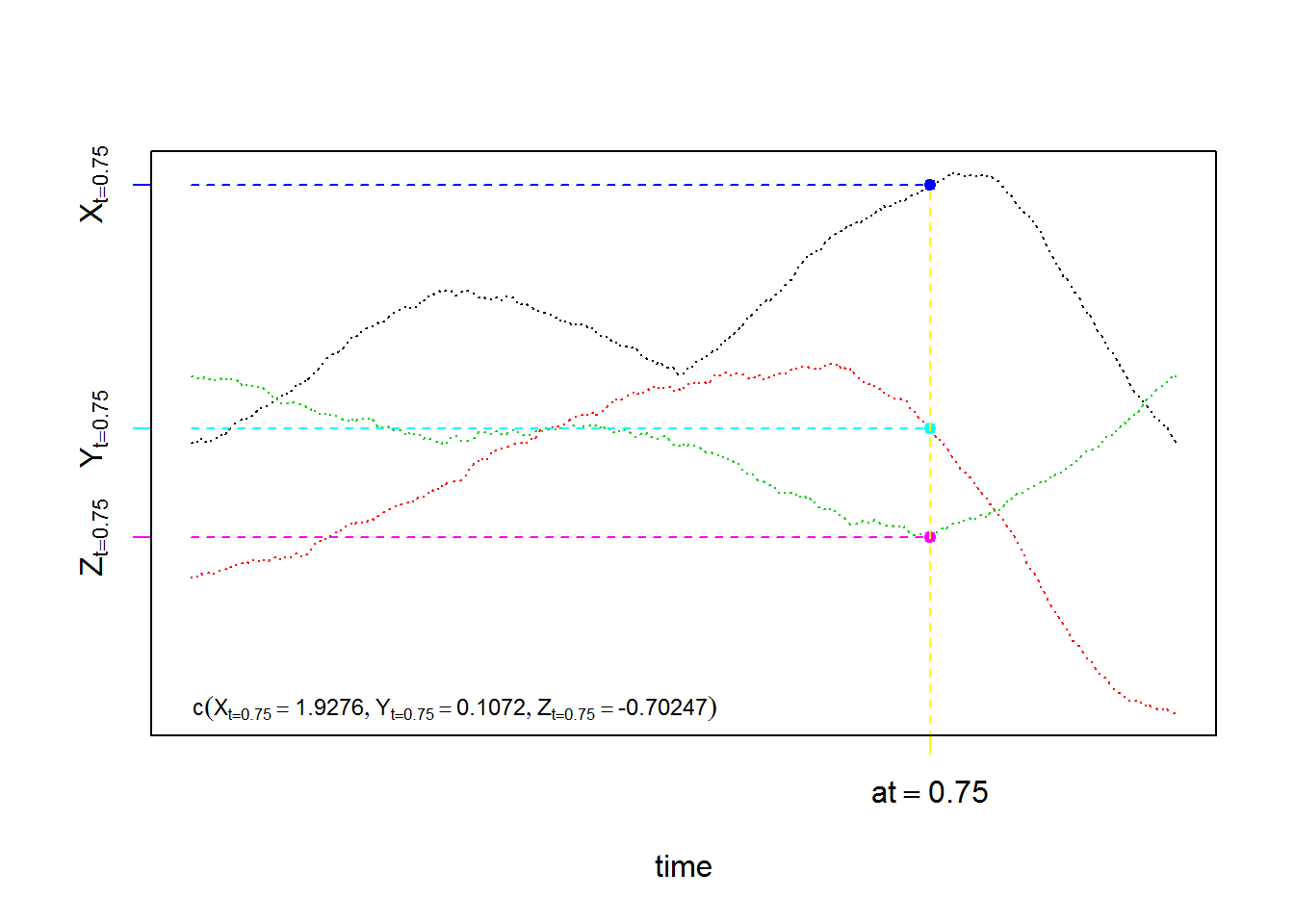For each SDE type and for each numerical scheme, the density of $$X_{t}|X_{0}=0,X_{T}=0$$, $$Y_{t}|Y_{0}=-1,Y_{T}=-2$$ and $$Z_{t}|Z_{0}=0.5,Z_{T}=0.5$$ process at time $$t=0.75$$ are reported using dsde3d() function, see e.g. Figure 15:

R> denM <- dsde3d(mod3,pdf="M",at =s)
R> denM

Marginal density of X(t-t0)|X(t0) = 0, X(T) = 0 at time t = 0.75

Data: x (999 obs.); Bandwidth 'bw' = 0.02448

x               f(x)
Min.   :1.5776   Min.   :0.0002
1st Qu.:1.7819   1st Qu.:0.0821
Median :1.9862   Median :0.7561
Mean   :1.9862   Mean   :1.2223
3rd Qu.:2.1906   3rd Qu.:2.3092
Max.   :2.3949   Max.   :3.5775

Marginal density of Y(t-t0)|Y(t0) = -1, Y(T) = -2 at time t = 0.75

Data: y (999 obs.); Bandwidth 'bw' = 0.01529

y                 f(y)
Min.   :-0.13891   Min.   :0.0003
1st Qu.: 0.00048   1st Qu.:0.0619
Median : 0.13988   Median :0.7422
Mean   : 0.13988   Mean   :1.7917
3rd Qu.: 0.27927   3rd Qu.:3.5669
Max.   : 0.41866   Max.   :5.9728

Marginal density of Z(t-t0)|Z(t0) = 0.5, Z(T) = 0.5 at time t = 0.75

Data: z (999 obs.); Bandwidth 'bw' = 0.03782

z                 f(z)
Min.   :-1.14685   Min.   :0.00017
1st Qu.:-0.83725   1st Qu.:0.05793
Median :-0.52766   Median :0.44584
Mean   :-0.52766   Mean   :0.80671
3rd Qu.:-0.21806   3rd Qu.:1.56529
Max.   : 0.09154   Max.   :2.33632  
R> plot(denM, main="Marginal Density")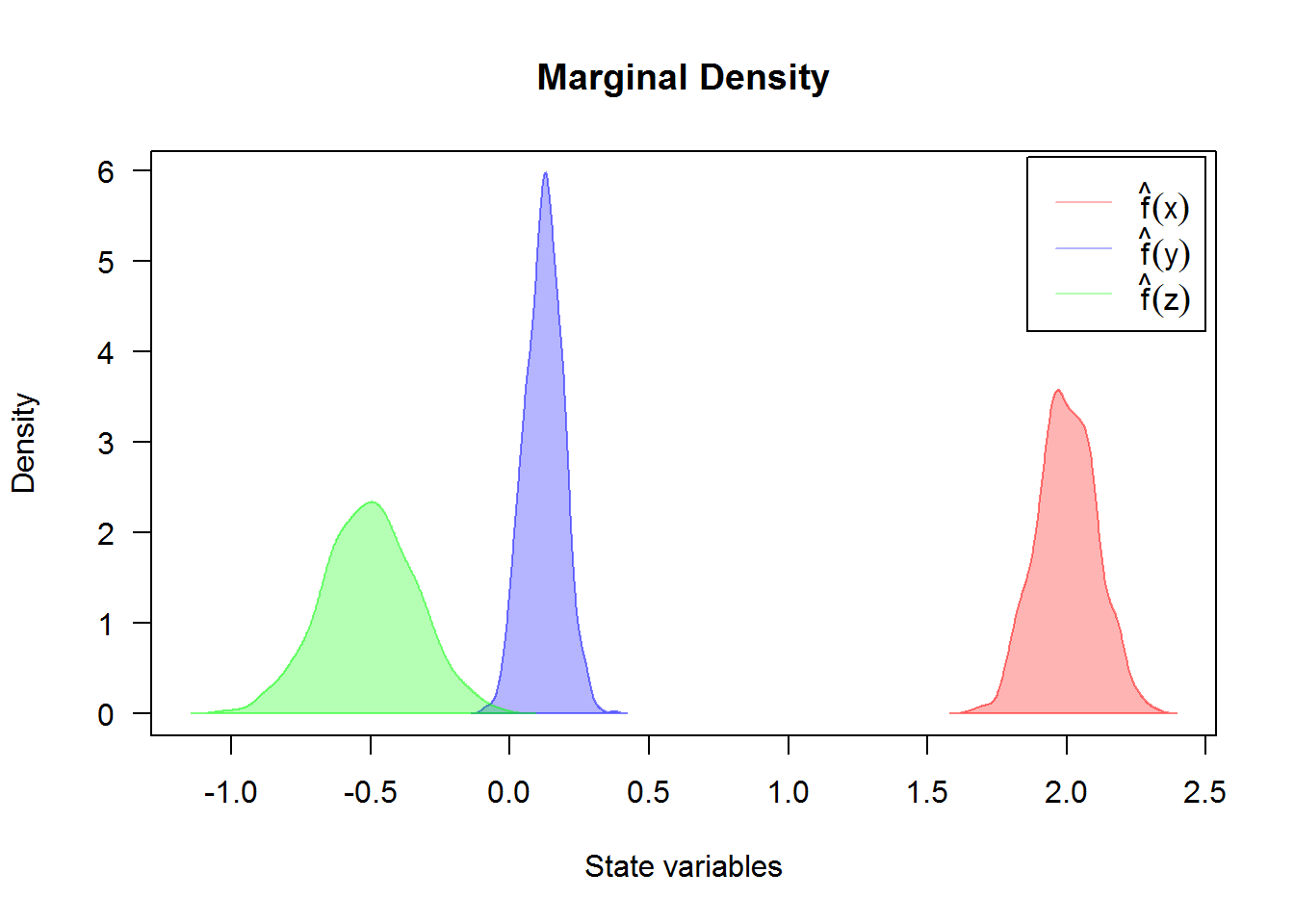For an approximate joint density for triplet $$X_{t},Y_{t},Z_{t}|X_{0}=0,Y_{0}=-1,Z_{0}=0.5,X_{T}=0,Y_{T}=-2,Z_{T}=0.5$$ at time $$t=0.75$$ (for more details, see package sm or ks.)

R> denJ <- dsde3d(mod3,pdf="J",at=0.75)
R> plot(denJ,display="rgl")